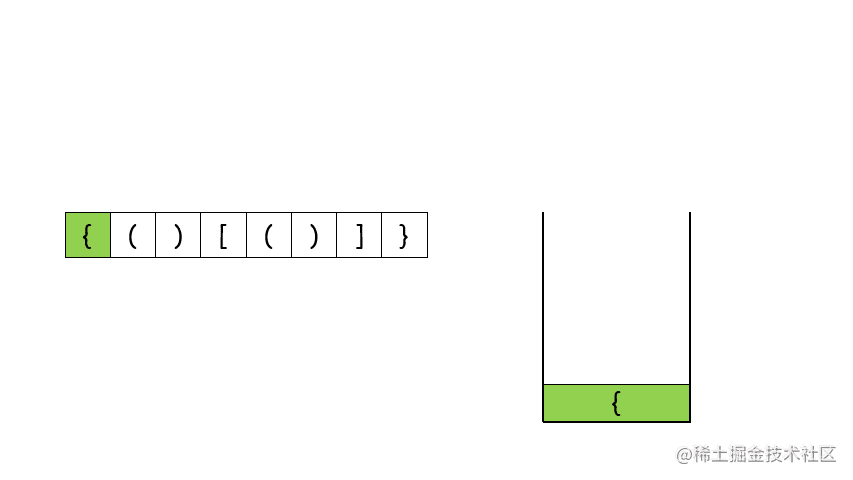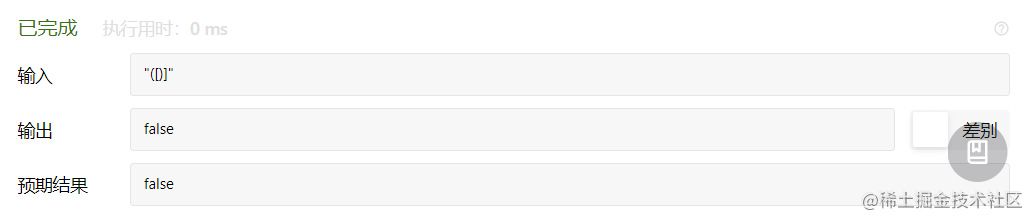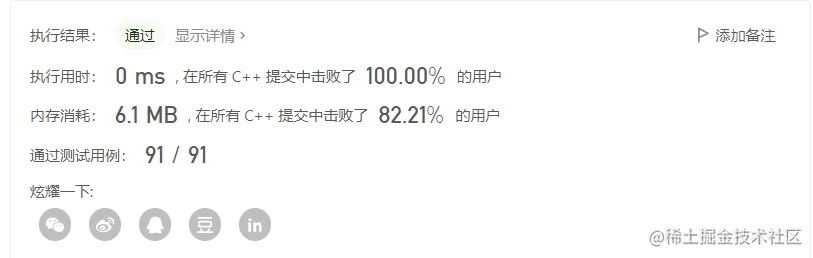# 算法题每日一练---第68天：有效的括号## 一、问题描述

1. 左括号必须用相同类型的右括号闭合。
2. 左括号必须以正确的顺序闭合。

## 二、题目要求

### 样例 1

``````输入： s = "()"

### 样例 2

``````输入： s = "([)]"

### 考察

``````1.字符串、栈、哈希
2.建议用时20~35min

## 三、问题分析

``````map<char,int>m={{'(',1},{'{',2},{'[',3},
{')',4},{'}',5},{']',6}};## 四、编码实现

``````class Solution {
public:
bool isValid(string s) {
int i,n=s.size();//初始化数据
bool flag=true;//一开始判断匹配成功
stack<char>k;
map<char,int>m={{'(',1},{'{',2},{'[',3},//哈希存储括号
{')',4},{'}',5},{']',6}};
for(i=0;i<n;i++)//循环判断
{
if(m[s[i]]<=3)//前三个直接存储
k.push(s[i]);

else if(!k.empty()&&m[s[i]]-3==m[k.top()])//后三个与栈顶匹配消除
k.pop();
else//匹配失败
{
flag=false;
break;
}
}
if(!k.empty())//如果栈不为空，那也匹配失败
flag=false;
return flag;//返回结果

}
};

## 五、测试结果